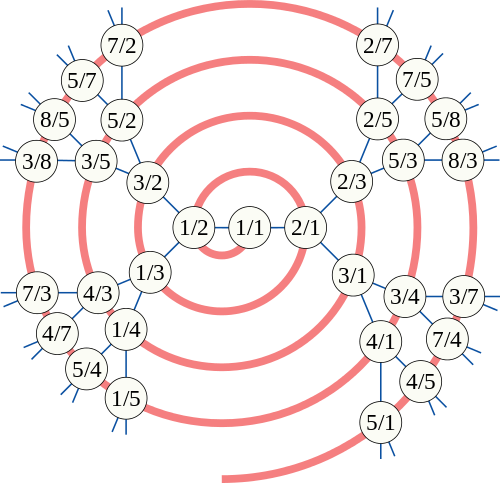# Enumerating the rationals

It is a well-known fact, attributed to Cantor, that the set of rationals is countably infinite or, equivalently, we can find a bijection between the rationals and integers. These are easy to construct, but typically quite ugly. If you want a closed-form bijection, you have to work harder, but it’s still possible.

My favourite enumeration of the (non-negative) rationals was discovered by Moshe Newman, and is a simple recurrence relation. More specifically, it is a very basic function f such that the sequence {0, f(0), f(f(0)), f(f(f(0))), …} contains each non-negative rational precisely once.$f(x) = \dfrac{1}{2 \lfloor x \rfloor - x + 1}$

If you’re not amazed by this, you should be. It generates the sequence {0/1, 1/1, 1/2, 2/1, 1/3, 3/2, 2/3, 3/1, 1/4, 4/3, 3/5, 5/2, 2/5, 5/3, …}, which can be proved to hit every non-negative rational exactly once (indeed, if it repeated a rational, it would become cyclic). Also, note that the denominator of one term is equal to the numerator of its successor, yielding the following sequence of positive integers:

0, 1, 1, 2, 1, 3, 2, 3, 1, 4, 3, 5, 2, 5, 3, … (sequence A002847 in OEIS)

This sequence, Stern’s diatomic sequence, is highly intriguing. We define the nth term to be fusc(n), so fusc(0) = 0 and fusc(1) = fusc(2) = 1, for example. There are several equivalent ways to define the fusc function:

• The rational counter description gives the second-order recurrence relation fusc(n) = fusc(n−1) − fusc(n−2) + 2 fusc(n−1) floor(fusc(n−2)/fusc(n−1)).
• There’s also the David-Monk-style pair of recurrence relations, fusc(2n) = fusc(n) and fusc(2n+1) = fusc(n) + fusc(n+1).
• The previous definition can be seen to be equivalent to the definition of fusc(n) as the number of ways of writing n as a sum of powers of two, such that no power of two appears more than twice.
• fusc(n) is also the number of odd binomial coefficients of the form$\binom{n-r}{r}$.

But yes, this is all pretty impressive. The sequence given by Newnham’s rational counter is a breadth-first traversal of the Calkin-Wilf tree, as shown below in this picture by David Eppstein:Each node$\dfrac{p}{q}$ has children$\dfrac{p}{p+q}$ and$\dfrac{p+q}{q}$. It is a routine exercise (which, I believe, has appeared on the British Mathematical Olympiad) to show that each positive rational appears precisely once in this binary tree.

This entry was posted in Uncategorized. Bookmark the permalink.

### 0 Responses to Enumerating the rationals

1.Thomas says:

Is the proof simple enough to write in a comment?

•Andrew Carlotti says:

The answer to that depends on how much maths you can take for granted.

•Andrew Carlotti says:

I’ve just realised this wasn’t the post I though it was when I wrote that comment. Whoops.

•apgoucher says:

With which other post did you confuse this? Surely not the punting in clearings of arbitrarily small Lebesgue measure?

•Andrew Carlotti says:

I mixed it up with http://cp4space.wordpress.com/2013/10/14/mathieu-groupoid/ where I thought that the answered problem at the beginning was an unanswered problem at the end. My confusion about the post itself was probably caused by an examples sheet, in which a very similar question appears at the end of the sheet.

•apgoucher says:

The proof that the Calkin-Wilf tree contains every rational precisely once? For injectivity, we know that if it contains the same rational twice, then Newnham’s rational counter would enter into a loop and therefore only reach finitely many rationals. Hence, we need only prove surjectivity.

Now, we can prove surjectivity by strong induction on p + q. Specifically, if p/q > 1, then it has (p – q)/q as its parent in the tree. Otherwise, if p/q < 1, then it has p/(q – p) as its parent. By strong induction, we are done.

•Thomas says:

I meant the proof that Newnham’s rational counter goes through all rationals.

2.drvitek says:

I don’t know about an appearance on the BMO, but this was problem A5 of the 2002 Putnam exam.

•apgoucher says:

Thanks. It may have been a current Advanced Mentoring Scheme problem, on recollection, and that indeed draws some material from the Putnam. Of course, this theorem is very well known, so it may have independently been entered into several olympiads.

3.Bill Gosper says:

To save you some effort, here are Neil Bickford’s Mathematica functions for the bijection.

calkinwilf[num_, denom_] := Block[{lis = {}, n = num, d = denom},
While[ n > 1 || d > 1, lis = Prepend[lis, Boole[n > d]];
If[n > d, n -= d, d -= n]]; lis]

wilfcalkin[bitlis_List] := Block[{n = 1, d = 1},
Do[If[bitlis[[i]] == 0, d += n, n += d], {i, 1, Length[bitlis]}];
Rational[n, d]]

newman2pos[num_, denom_] :=
FromDigits[Prepend[calkinwilf[num, denom], 1], 2]

newman2pos[frac_] := newman2pos[Numerator[frac], Denominator[frac]]

pos2newman[pos_] := wilfcalkin[IntegerDigits[pos, 2][[2 ;; -1]]]

E.g., newman2pos /@ Convergents[√3, 9]
{1, 3, 13, 29, 109, 237, 877, 1901, 7021}

FullSimplify[FindSequenceFunction[%, n]]
(-40 + 2^(3 n/2) (13 + 12 Sqrt + (-1)^n (13 – 12 Sqrt)))/56

% /. n -> 22
3988183917

pos2newman[%]^2 – 3
1/318907548961

4.Andrew Carlotti says:

I don’t see how this definition works:
“The previous definition can be seen to be equivalent to the definition of fusc(n) as the number of ways of writing n as a sum of powers of two, such that no power of two appears more than twice.”
Could you explain?

•Sam Cappleman-Lynes says:

By my reckoning, fusc(n) should be the number of ways of writing n-1 as a sum of powers of 2, each power appearing no more than twice.

•Andrew Carlotti says:

That makes a lot more sense – I think I can prove that.